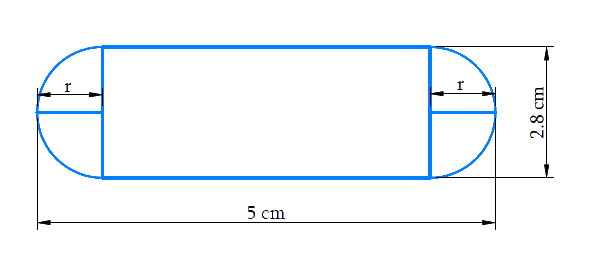# Ex.13.2 Q3 Surface Areas and Volumes Solution - NCERT Maths Class 10

Go back to  'Ex.13.2'

## Question

A Gulab jamun contains sugar syrup up to about $$30 \%$$ of its volume. Find approximately how much syrup would be found in $$45$$ Gulab jamuns, each shaped like a cylinder with two hemispherical ends with length $$5\,\rm{cm}$$ and diameter $$2.8\,\rm{cm}$$ (see Fig. 13.15).

Video Solution
Surface Areas And Volumes
Ex 13.2 | Question 3

## Text Solution

What is known?

Each Gulab jamun’s shape is like a cylinder with $$2$$ hemispherical ends with length $$5\rm{cm}$$ and diameter $$2.8\rm{cm.}$$
Each Gulab jamun contains sugar syrup up to about $$30\%$$ of its volume.

What is unknown?

Volume of sugar syrup in $$45$$ Gulab jamuns (approximately)

Reasoning:

Draw the figure to visualize the shape of Gulab jamunFig. 13.15

From the figure it’s clear that

Length of cylindrical part $$=$$ length of a Gulab jamun $$- {\rm{ }}2 \times$$ radius of the hemispherical part

Also,

Diameter of Gulab jamun $$=$$ diameter of cylindrical part

Radius of cylindrical part $$=$$ radius of hemispherical part

In order to find volume of sugar syrup in $$45$$ Gulab jamun we find volume of Gulab jamun

Using the statement

The volume of the solid formed by joining 2 basic solids will actually be sum of the volumes of its constituents.

Volume of $$1$$ Gulab jamun $$=$$ volume of cylindrical part $$+$$ volume of the $$2$$ hemispherical parts

Since, it’s given a Gulab jamun contains sugar syrup up to about $$30\%$$ of its volume. We make an assumption to every Gulab jamun contains sugar syrup at $$30\%$$ of its volume to simplify calculation

Volume of sugar syrup $$= 30\%$$ of volume of $$45$$ Gulab jamun.

Steps:

Diameter of the Gulab jamun,$$d = 2.8 \rm cm$$

Radius of cylindrical part $$=$$ radius of hemispherical part\begin{align} = r = \frac{{2.8 \rm cm}}{2} = 1.4cm\end{align}

Length of cylindrical part,$$h = 5 \rm cm - 2 \times 1.4cm = 2.2cm$$

Volume of $$1$$ Gulab jamun $$=$$ volume of cylindrical part$$+ {\rm{ 2}} \times$$ volume of the hemispherical parts

\begin{align}&= \pi {r^2}h + 2 \times \frac{2}{3}\pi {r^3}\\&= \pi {r^2}h + \frac{4}{3}\pi {r^3}\\&= \pi {r^2}\left( {h + \frac{4}{3}r} \right)\\&= \begin{bmatrix} \frac{{22}}{7} \times 1.4 \rm cm \times 1.4cm \\ \times \begin{pmatrix} 2.2 \rm cm + \frac{4}{3} \\ \times 1.4 \rm cm \end{pmatrix} \end{bmatrix} \\&= \begin{bmatrix} \frac{{22}}{7} \times 1.4 \rm cm \times \\ 1.4 \rm cm \times \frac{{122.2}}{3} \rm cm \end{bmatrix} \\&= \frac{{75.152}}{3} \rm c{m^3}\end{align}

Volume of $$45$$ Gulab jamuns $$= 45 \times$$ volume of $$1$$ Gulab jamun

\begin{align}&= 45 \times \frac{{75.152}}{3} \rm c{m^3}\\&= 15 \times 75.152 \rm c{m^3}\\&= 1127.28 \rm c{m^3}\end{align}

Volume of sugar syrup in $$45$$ Gulab jamuns$$= 30\%$$   of volume of $$45$$ Gulab jamun

\begin{align}&= \frac{{30}}{{100}} \times 1127.28 \rm c{m^{^3}}\\ &= 338.184 \rm c{m^3}\\ &= 338{\rm{ c{m^3 }}}({\text{approximately}})\end{align}

Learn from the best math teachers and top your exams

• Live one on one classroom and doubt clearing
• Practice worksheets in and after class for conceptual clarity
• Personalized curriculum to keep up with school